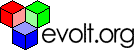Work

# Coldfusion Strings Masks And Filters

Rated 4.05 (Ratings: 4)

#### Want more?#### Joshua Olson

Member info

User since: 21 Sep 2000

Articles written: 3

Purpose: Applies generic masks to filtered strings

Method: brute force

##### Attributes:

• `value` - the value to which we apply the mask
• `result` - variable in which to store the answer, defaults to "out"
• `mask` - mask to apply to the value
• `filter` - prefilter of value

No parameters are required, though not specifying anything is boring.

`filter` accepts "alpha", "numeric", "alphanumeric" and is a prefilter to the string. For example, the string "a1b2-c3c4" would be processed as the following with the different filters:

• `none`: "a1b2-c3d4"
• `alpha`: "abcd"
• `numeric`: "1234"
• `alphanumeric`: "a1b2c3d4"

The filtered string would *then* be applied to the mask. The mask

entities are as follows:

• `_` character unchanged
• `0` character is unchanged for numerics and forced to zero for all others
• `9` character is unchanged for numerics and forced to empty for all others
• `a` character is forced to lower case, all others are left as is
• `A` character is forced to upper case, all others are left as is
• `b` character is forced to lower case, numerics are forces to empty
• `B` character is forced to upper case, numerics are forces to empty
• `\` following character is literal

Usage:

`<cf_filtermask value="123abc" mask="___ ___" result="license_plate">`

returns: 123 abc

`<cf_filtermask value="ab 1sf 2 sd 3 s 45" mask="\_0+\0b999999" filter="numeric">`

returns: _1+0345

`<cf_filtermask value="APPL9E" mask="Bbbbbb \B">`

returns: Apple B

`<cf_filtermask value="706-555-1212ext123" mask="(___)___-____"`

`filter="numeric">`

and

`<cf_filtermask value="7065551212" mask="(___)___-____" filter="numeric">`

return: (706)555-1212

The last example is why I made it in the first place.

`<cfsilent><cfparam name="attributes.value" default=""><cfparam name="attributes.result" default="out"><cfparam name="attributes.mask" default=""><cfparam name="attributes.filter" default=""><cfset mask = attributes.mask><cfset t_value = attributes.value><cfif attributes.filter IS "numeric">  <cfset t_value = REReplace(t_value, "[^[:digit:]]", "", "ALL")></cfif><cfif attributes.filter IS "alpha">  <cfset t_value = REReplace(t_value, "[^[:alpha:]]", "", "ALL")></cfif><cfif attributes.filter IS "alphanumeric">  <cfset t_value = REReplace(t_value, "[^[:alnum:]]", "", "ALL")></cfif><cfset value = ""><cfset pos = "1"><cfset literal = "0"><cfloop index="i" from="1" to="#Len(mask)#">  <cfset character = Mid(mask, i, 1)>  <cfif literal>    <cfset value = value & character>    <cfset literal = "0">  <cfelse>    <cfif Len(t_value) GTE pos>      <cfset char_at_pos = Mid(t_value, pos, 1)>    <cfelse>      <cfset char_at_pos = "">    </cfif>    <cfif character IS "9">      <cfif isNumeric(char_at_pos)>        <cfset value = value & Val(char_at_pos)>      </cfif>      <cfset pos = pos + "1">    <cfelseif character IS "0">      <cfset value = value & Val(char_at_pos)>      <cfset pos = pos + "1">    <cfelseif character IS "_">      <cfset value = value & char_at_pos>      <cfset pos = pos + "1">    <cfelseif Find("A", character)>      <cfset value = value & UCase(char_at_pos)>      <cfset pos = pos + "1">    <cfelseif Find("a", character)>      <cfset value = value & LCase(char_at_pos)>      <cfset pos = pos + "1">    <cfelseif Find("b", character)>      <cfif NOT isNumeric(char_at_pos)>        <cfset value = value & LCase(char_at_pos)>      </cfif>      <cfset pos = pos + "1">    <cfelseif Find("B", character)>      <cfif NOT isNumeric(char_at_pos)>        <cfset value = value & UCase(char_at_pos)>      </cfif>      <cfset pos = pos + "1">    <cfelseif Find("\", character)>      <cfset literal = "1">    <cfelse>      <cfset value = value & character>    </cfif>  </cfif> <!--- if literal ---></cfloop><cfset t = SetVariable("caller.#attributes.result#", value)></cfsilent>`

The access keys for this page are: ALT (Control on a Mac) plus:Evolt.org is an all-volunteer resource for web developers made up of a discussion list, a browser archive, and member-submitted articles. This article is the property of its author, please do not redistribute or use elsewhere without checking with the author.